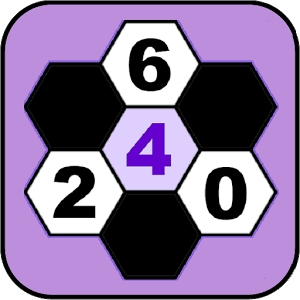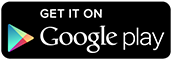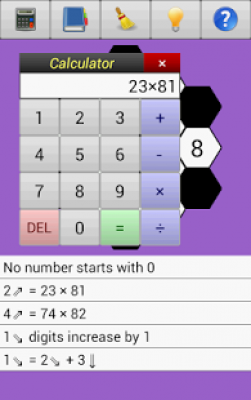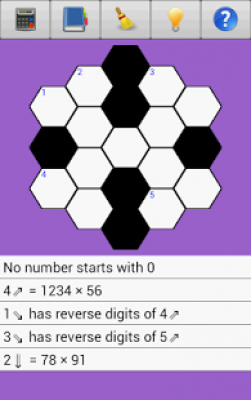Are you RikkiGames? AppZUMBi generated this app from content on Google Play.
Click here to claim Math Hexagon Puzzles.This is a FREE app.

Developer:

App Type:

Categories:

Content Rating:

Quick Description:
Math Hexagon Puzzles is a free set of cross-figure logic puzzles (the numerical version of a crossword).

Math Hexagon Puzzles is a free set of cross-figure logic puzzles (the numerical version of a crossword), using a hexagonal grid instead of the usual crossword puzzle grid. Clues are given to each puzzle and the player fills in blank spaces with numbers making sure they meet the criteria of the clues.

There are 4 different sets of puzzles ranging from Easy puzzles, designed to help ease players into the process of completing the math puzzles, and finishing with Very Hard puzzles which require more time to think through and solve. The player has to achieve a certain number of puzzles before they can unlock later sets of puzzles.

Each puzzle has clues, calculator, list of square numbers, list of cubed numbers and a list of prime numbers to assist the player in completion of the puzzles. The player also starts with 5 hints and receives extra ones when a level is completed to help solve the puzzles which are causing a challenge.

This app follows the same concept as the Math Puzzle Challenge app, (also published by RikkiGames), except that the puzzles are more straightforward.

What makes this set of puzzles more interesting is the challenge of working with a hexagonal grid instead of the usual square grid. Clues go diagonally up, diagonally down and down instead of the usual across and down. This means that a digit could be part of three different numbers. The hexagonal crossword grids lend themselves to some interesting patterns using reflective and rotational symmetry. The puzzle grids are three different sizes. The lengths of the hexagonal grids used for this set of puzzles is 2, 3 and 4 allowing for 7, 19 and 37 blank spaces or digits.

There are a total of 60 puzzles with four levels of difficulty, Easy, Normal, Hard and Very Hard

List of puzzle difficulties and number of puzzles:
- Easy has 14 puzzles
- Normal has 22 puzzles
- Hard has 12 puzzles
- Very Hard has 12 puzzles

Math Hexagonal Puzzles can also help improve your math skills and knowledge of number properties. How do you know if a number is divisible by 2, 3, 4 or 11? What are the only digits that a square number can end with? What is the largest number whose square is less than 1000? What are the possible values that the first digit of a 4 digit number have if it is 5 times a 3 digit number? What are the only digits which a prime number can end with? As you work through the puzzles, you may start to discover the answers to these questions and other number properties, if you do not already know them.
App Email:
App Website: http://rikkigames.com/

Share
Share on Tumblr
Download Math Hexagon Puzzles from these app stores today.Screen Shots of Math Hexagon Puzzles